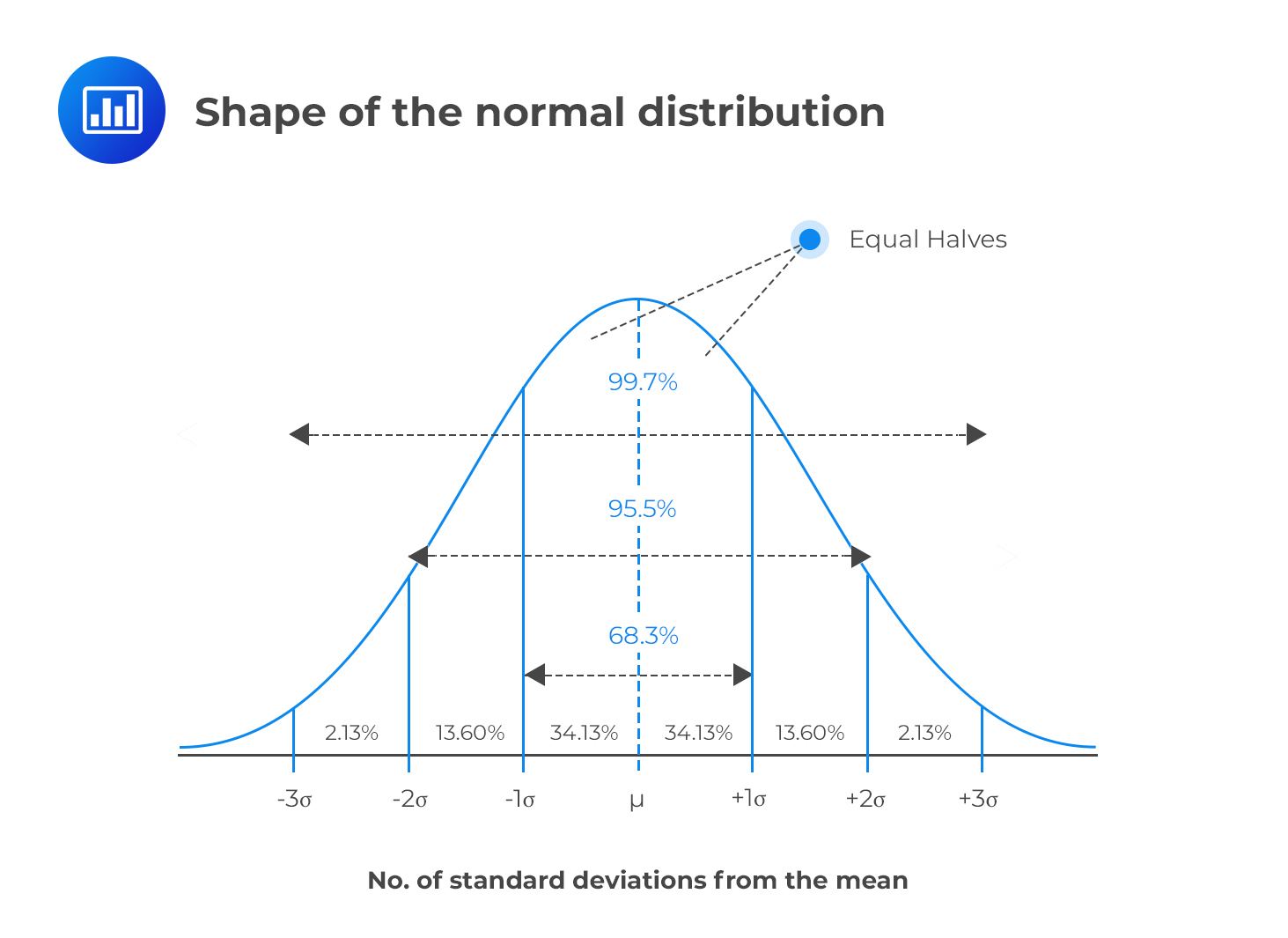Limited Time Offer: Save 10% on all 2022 Premium Study Packages with promo code: BLOG10# Normal Distribution

A random variable is said to have the normal distribution (Gaussian curve) if its values make a smooth curve that assumes a “bell shape.” A normal variable has a mean $$μ$$, pronounced as “mu,” and a standard deviation $$σ$$, pronounced as “sigma.” All normal distributions have a distinguishable bell shape regardless of the mean, variance, and standard deviation.A normal distribution has certain properties that make it a useful tool in the world of finance.

1. Its shorthand notation is $$X \sim N(\mu , \sigma^2)$$. This is read as “the random variable X has a normal distribution with mean $$μ$$ and variance $$σ^2$$.”
2. It has a symmetric shape, meaning it can be cut into two halves that are mirror images of each other; as such, skewness = 0.
3. Kurtosis = 3. Remember that kurtosis is a measure of flatness and excess kurtosis is measured relative to 3, the “normal kurtosis.”
4. The mean, mode, and median are all equal and lie directly in the middle of the distribution.
5. If $$X \sim N(\mu_x, \sigma^2_x)$$ and $$Y\sim N(\mu_y, \sigma^2_y)$$, then the two variables combined also have a normal distribution.
6. The standard deviation measures the distance from the mean to the point of inflection, which is the point where the curve changes an “upside-down-bowl” shape to a “right-side-up-bowl” shape.
7. Probabilities follow the empirical rule. About 68% of the total values lie within one standard deviation of the mean, 95% of the values lie within two standard deviations of the mean, and 99.7% lie within three standard deviations of the mean.

## Area Under the Normal Distribution Curve

To determine the probability that a random variable $$X$$ lies between two points $$a$$ and $$b$$:

$$P\left( a < X < b \right) =\int _{ a }^{ b }{ f\left( x \right)dx }$$

The normal distribution is very important in statistical analysis, especially because of the central limit theorem. The theorem asserts that any distribution becomes distributed normally when the number of variables is sufficiently large. For instance, the binomial distribution tends to “change” into the normal distribution with mean $$nθ$$ and variance $$nθ (1 – θ)$$.

### Question

Which of the following is least likely a property of normal distribution?

1. The excess kurtosis is equal to 3.
2. The mean, mode, and median are all equal.
3. About 68% of the total values lie within one standard deviation of the mean.

Solution

The corect answer is A.

The normal distribution has an excess kurtosis of 0. Excess kurtosis is a value above kurtosis of 3. Since the kurtosis of the normal distribution is 3, the excess kurtosis of 0.

B and C are incorrect. They are the true properties of the normal distribution.

Shop CFA® Exam Prep

Offered by AnalystPrepLevel I
Level II
Level III
All Three Levels
Featured Shop FRM® Exam PrepFRM Part I
FRM Part II
FRM Part I & Part II
Learn with Us

Subscribe to our newsletter and keep up with the latest and greatest tips for success
Shop Actuarial Exams PrepExam P (Probability)
Exam FM (Financial Mathematics)
Exams P & FM
Shop GMAT® Exam PrepComplete CourseSergio Torrico
2021-07-23
Excelente para el FRM 2 Escribo esta revisión en español para los hispanohablantes, soy de Bolivia, y utilicé AnalystPrep para dudas y consultas sobre mi preparación para el FRM nivel 2 (lo tomé una sola vez y aprobé muy bien), siempre tuve un soporte claro, directo y rápido, el material sale rápido cuando hay cambios en el temario de GARP, y los ejercicios y exámenes son muy útiles para practicar.diana
2021-07-17
So helpful. I have been using the videos to prepare for the CFA Level II exam. The videos signpost the reading contents, explain the concepts and provide additional context for specific concepts. The fun light-hearted analogies are also a welcome break to some very dry content. I usually watch the videos before going into more in-depth reading and they are a good way to avoid being overwhelmed by the sheer volume of content when you look at the readings.Kriti Dhawan
2021-07-16
A great curriculum provider. James sir explains the concept so well that rather than memorising it, you tend to intuitively understand and absorb them. Thank you ! Grateful I saw this at the right time for my CFA prep.nikhil kumar
2021-06-28
Very well explained and gives a great insight about topics in a very short time. Glad to have found Professor Forjan's lectures.Marwan
2021-06-22
Great support throughout the course by the team, did not feel neglectedBenjamin anonymous
2021-05-10
I loved using AnalystPrep for FRM. QBank is huge, videos are great. Would recommend to a friendDaniel Glyn
2021-03-24
I have finished my FRM1 thanks to AnalystPrep. And now using AnalystPrep for my FRM2 preparation. Professor Forjan is brilliant. He gives such good explanations and analogies. And more than anything makes learning fun. A big thank you to Analystprep and Professor Forjan. 5 stars all the way!michael walshe
2021-03-18
Professor James' videos are excellent for understanding the underlying theories behind financial engineering / financial analysis. The AnalystPrep videos were better than any of the others that I searched through on YouTube for providing a clear explanation of some concepts, such as Portfolio theory, CAPM, and Arbitrage Pricing theory. Watching these cleared up many of the unclarities I had in my head. Highly recommended.